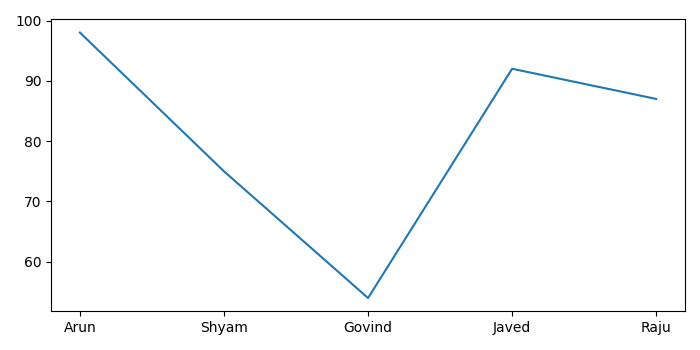# Plot data from CSV file with Matplotlib

To extract CSV file for specific columns to list in python, we can use Pandas read_csv() method.

## Steps

• Make a list of columns that have to be extracted.
• Use read_csv() method to extract the CSV file data into a data frame.
• Print the exracted data.
• Plot the data frame using plot() method.
• To display the figure, use show() method.

## Example

import pandas as pd
from matplotlib import pyplot as plt
plt.rcParams["figure.figsize"] = [7.00, 3.50]
plt.rcParams["figure.autolayout"] = True
columns = ["Name", "Marks"]
plt.show()